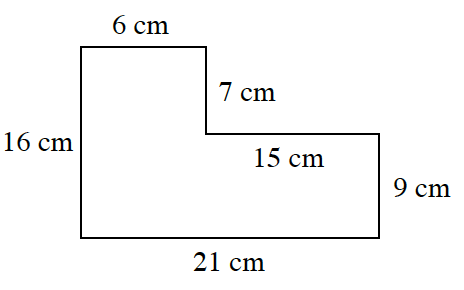### Home > CCG > Chapter 1 > Lesson 1.1.5 > Problem1-44

1-44.

Angela had a rectangular piece of paper and then cut a rectangle out of a corner as shown at right. Find the area and perimeter of the resulting shape.Perimeter is the distance around the exterior of a figure.

Area is the number of 'squares' needed to cover the interior of a figure.

Divide this shape into smaller rectangles to find the area.

$6+7+15+9+21+16=74$ cm